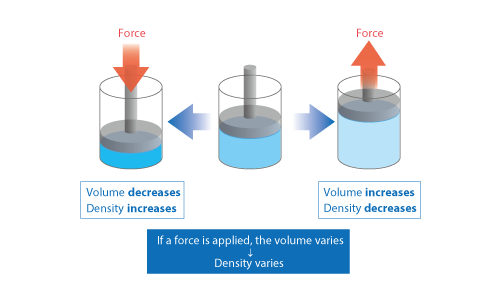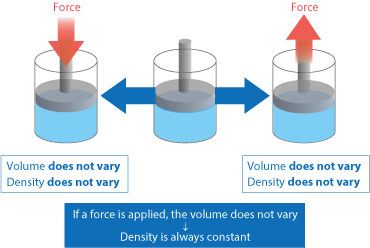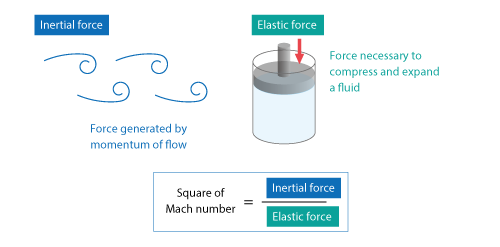# Want to Know More! Basics of Thermo-Fluid Analysis 17: Chapter 3 Flow 3.3.2 Compressibility and incompressibility (2)

#### 3.3.2 Compressibility and incompressibility (2)

This column describes behaviors of compressible fluids and incompressible fluids more concretely.

For compressible fluids, let us see variation of fluid volume. If a cylinder is filled with a compressible fluid and a force is applied on a piston as shown in Figure 3.20, the fluid volume decreases or increases. Because mass of the fluid in the cylinder is constant, variation of its volume causes variation of its density .Figure 3.20 Characteristics of compressible fluid

On the other hand, volume of incompressible fluids does not vary. That is, even if a force is applied on the piston as shown in Figure 3.21, the fluid volume does not decrease or increase.Figure 3.21 Characteristics of incompressible fluids

In a system where an incompressible fluid inflows and outflows as shown in Figure 3.22, the fluid in the system is pushed out by the inflow fluid. This means that the inflow volume and the outflow volume must be equal. If they are not equal, an incompressible fluid is continuously crammed into or removed from a space of a certain volume. This means the volume of the incompressible fluid varies. Then, you will find it contradictory.Figure3.22 A system with inflow and outflow

In thermo-fluid analyses , you need to select whether a fluid is compressible or incompressible. Rigorously speaking, real fluids are compressible; however in incompressible fluid analyses, calculation settings are simpler and calculation time is shorter. This is why a fluid with a small Mach number and little influence of compressibility is generally treated as an incompressible fluid for calculation.

Want to know more  What is Mach number?
The square of a Mach number shows the ratio of the inertial force of a flow to the elastic force of a fluid.

As shown in Figure 3.23, inertial force is generated by the momentum of a flow, and elastic force is necessary to compress and expand a fluid. So, we can see that a Mach number shows how much a fluid is compressed by the momentum of its flow.Figure 3.23 Physical definition of Mach number

Flow of a fluid with a large Mach number (fast flow) has an inertial force greater than the elastic force of the fluid. Therefore, the fluid is greatly compressed by its flow and the influence of compressibility cannot be ignored.

On the other hand, flow of a fluid with a small Mach number (slow flow) has an inertial force smaller than the elastic force of the fluid. In this case, the degree of fluid compression is enough small to ignore the influence of compressibility.

For thresholds of Mach number and the meanings of the values, see  Want to Know More   in the previous column.